# SQL面试经典50题

SQL语句的执行顺序：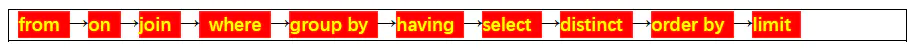create database school;
use school;


create table student(
s_id varchar(10),
s_name varchar(20),
s_age date,
s_sex varchar(10)
);

create table course(
c_id varchar(10),
c_name varchar(20),
t_id varchar(10)
);

create table teacher (
t_id varchar(10),
t_name varchar(20)
);

create table score (
s_id varchar(10),
c_id varchar(10),
score varchar(10)
);


insert into student (s_id, s_name, s_age, s_sex)
values  ('01' , '赵雷' , '1990-01-01' , '男'),
('02' , '钱电' , '1990-12-21' , '男'),
('03' , '孙风' , '1990-05-20' , '男'),
('04' , '李云' , '1990-08-06' , '男'),
('05' , '周梅' , '1991-12-01' , '女'),
('06' , '吴兰' , '1992-03-01' , '女'),
('07' , '郑竹' , '1989-07-01' , '女'),
('08' , '王菊' , '1990-01-20' , '女');

insert into course (c_id, c_name, t_id)
values  ('01' , '语文' , '02'),
('02' , '数学' , '01'),
('03' , '英语' , '03');

insert into teacher (t_id, t_name)
values  ('01' , '张三'),
('02' , '李四'),
('03' , '王五');

insert into score (s_id, c_id, score)
values  ('01' , '01' , 80),
('01' , '02' , 90),
('01' , '03' , 99),
('02' , '01' , 70),
('02' , '02' , 60),
('02' , '03' , 80),
('03' , '01' , 80),
('03' , '02' , 80),
('03' , '03' , 80),
('04' , '01' , 50),
('04' , '02' , 30),
('04' , '03' , 20),
('05' , '01' , 76),
('05' , '02' , 87),
('06' , '01' , 31),
('06' , '03' , 34),
('07' , '02' , 89),
('07' , '03' , 98);create table total(
select a.s_id as s_id,a.s_name as s_name,a.s_age as s_age,a.s_sex as s_sex,
b.c_id as c_id,b.score as score,c.t_id as t_id,d.t_name as t_name
from student a
left join
score  b on a.s_id=b.s_id
left join
course c on b.c_id=c.c_id
left join
teacher d on c.t_id=d.t_id
);
select * from total;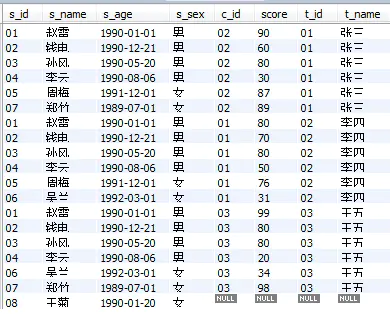# ***1、查询"01"课程比"02"课程成绩高的学生的信息及课程分数

select a.s_id as s_id,score1,score2 from
(select s_id, score as score1 from score where c_id='01') a
inner join
(select s_id, score as score2 from score where c_id='02') b
on a.s_id=b.s_id
where score1>score2;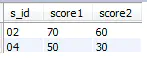# 2、查询"01"课程比"02"课程成绩低的学生的信息及课程分数

select a.s_id as s_id,score1,score2 from
(select s_id, score as score1 from score where c_id='01') a
inner join
(select s_id, score as score2 from score where c_id='02') b
on a.s_id=b.s_id
where score1<score2;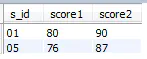# 3、查询平均成绩大于等于60分的同学的学生编号和学生姓名和平均成绩

select student.s_id as s_id,student.s_name as s_name,b.avg_score as avg_score from student
right join
(select s_id,avg(score) as avg_score from score
group by s_id having avg_score>60) b
on student.s_id=b.s_id;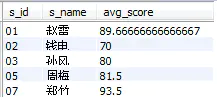# 4、查询平均成绩小于60分的同学的学生编号和学生姓名和平均成绩

select student.s_id as s_id,student.s_name as s_name,b.avg_score as avg_score from student
right join
(select s_id,avg(score) as avg_score from score
group by s_id having avg_score<60) b
on student.s_id=b.s_id;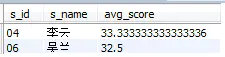# 5、查询所有同学的学生编号、学生姓名、选课总数、所有课程的总成绩

select s_id, s_name, count(c_id) as c_num, sum(score) as total_score
from total
group by s_id ;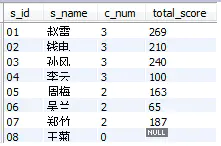# 6、查询"李"姓老师的数量

select count(t_name) from teacher
where t_name like '李%';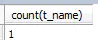# 7、查询学过"张三"老师授课的同学的信息

select distinct s_id,s_name,s_age,s_sex
from total
where t_name='张三';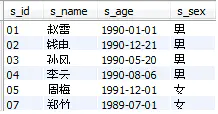# 8、查询没学过"张三"老师授课的同学的信息

select * from student
where s_id not in
(select distinct s_id
from total
where t_name='张三');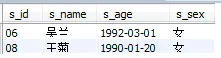# 9、查询学过编号为"01"并且也学过编号为"02"的课程的同学的信息

select * from student
where s_id in
(select s_id from score where c_id='01')
and s_id in
(select s_id from score where c_id='02');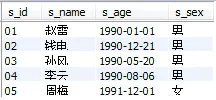# 10、查询学过编号为"01"但是没有学过编号为"02"的课程的同学的信息

select * from student
where s_id in
(select s_id from score where c_id='01')
and s_id not in
(select s_id from score where c_id='02');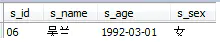# 11、查询没有学全所有课程的同学的信息

select s_id, s_name, s_age, s_sex from total
group by s_id having count(c_id) <3 ;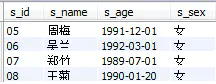# 12、查询至少有一门课与学号为"01"的同学所学相同的同学的信息

select * from student
where s_id in
(select distinct s_id from score
where c_id in
(select c_id from score where s_id='01'));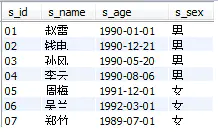# ***13、查询和"01"号的同学学习的课程完全相同的其他同学的信息

select * from student
where s_id in
(select s_id from
(select score.s_id,a.c_id from
(select c_id from score where s_id='01') a
inner join  score
on a.c_id=score.c_id) b
where s_id<>'01'
group by s_id having count(c_id)=
(select count(c_id) from score where s_id='01'));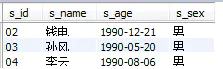# 14、查询没学过"张三"老师讲授的任一门课程的学生姓名

select s_id,s_name from student
where s_id not in
(select distinct s_id from total
where t_name='张三');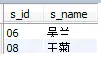# 15、查询两门及其以上不及格课程的同学的学号，姓名及其平均成绩

select a.s_id,student.s_name,b.avg_score from
(select s_id from score
where score<60
group by s_id having count(*)>=2) a
left join
student on a.s_id=student.s_id
left join
(select s_id,avg(score) as avg_score
from score
group by s_id) b
on a.s_id=b.s_id;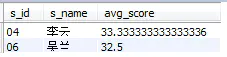# 16、检索"01"课程分数小于60，按分数降序排列的学生信息

select a.s_id,student.s_name,student.s_age,student.s_sex,a.score from
(select s_id,score from score
where c_id='01' and score<60
order by score desc) a
left join student on a.s_id=student.s_id;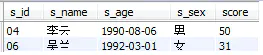# ***17、按平均成绩从高到低显示所有学生的所有课程的成绩以及平均成绩

select s_id as '学号',
sum(case c_id when '01' then score else 0 end) as '语文',
sum(case c_id when '02' then score else 0 end) as '数学',
sum(case c_id when '03' then score else 0 end) as '英语',
avg(score) as '平均成绩'
from score
group by s_id
order by '平均成绩' desc;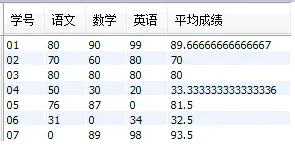# *****18、查询各科成绩最高分、最低分和平均分：以如下形式显示：课程ID，课程name，最高分，最低分，平均分，及格率，中等率，优良率，优秀率

## ----及格为>=60，中等为：70-80，优良为：80-90，优秀为：>=90

select a.c_id as '课程ID',course.c_name as '课程name',
max(a.score) as '最高分',min(a.score) as '最低分',
cast(avg(a.score) as decimal(5,2)) as '平均分',
concat(cast(sum(pass)/count(*)*100 as decimal(5,2)),'%') as '及格率',
concat(cast(sum(medi)/count(*)*100 as decimal(5,2)),'%') as '中等率',
concat(cast(sum(good)/count(*)*100 as decimal(5,2)),'%') as '优良率',
concat(cast(sum(excellent)/count(*)*100 as decimal(5,2)),'%') as '优秀率' from
(select * ,
case when score>=60 then 1 else 0 end as pass,
case when score>=70 and score<80 then 1 else 0 end as medi,
case when score>=80 and score<90 then 1 else 0 end as good,
case when score>=90 then 1 else 0 end as excellent
from score) a
left join course on a.c_id=course.c_id
group by a.c_id;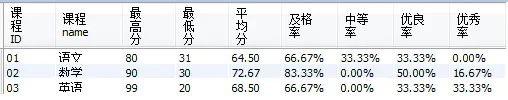# 19、按各科成绩进行排序，并显示排名

select a.*,@rank:=@rank+1 as rank from
(select c_id,sum(score) as '成绩' from score
group by c_id order by sum(score) desc) a,
(select @rank:=0) b;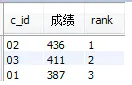# 20、查询学生的总成绩并进行排名

select a.*,@rank:=@rank+1 as rank from
(select s_id,sum(score) as '总成绩' from score
group by s_id order by sum(score) desc) a,
(select @rank:=0) b;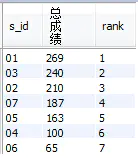# 21、查询不同老师所教不同课程平均分从高到低显示

select t_id,t_name,c_id,avg(score) as avg_score
from total
group by t_id,c_id
order by avg_score desc;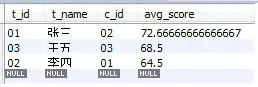# ****22、查询所有课程的成绩第2名到第3名的学生信息及该课程成绩

select c_id,s_id,score from score
group by c_id,s_id order by c_id,score desc;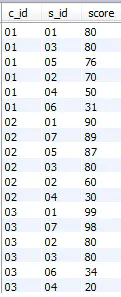select *,if(@pa=a.c_id,@rank:=@rank+1,@rank:=1) AS rank,@pa:=a.c_id
from
(select c_id,s_id,score from score
group by c_id,s_id order by c_id,score desc) a,
(select @rank:=0,@pa:=NULL) b;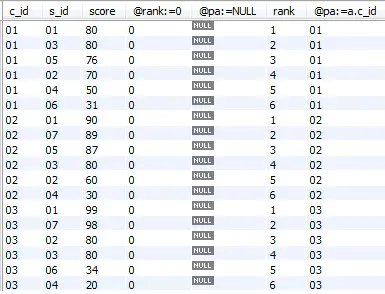select result.c_id,result.s_id,result.score,
student.s_name,student.s_age,student.s_sex from
(select *,if(@pa=a.c_id,@rank:=@rank+1,@rank:=1) AS rank,@pa:=a.c_id
from
(select c_id,s_id,score from score
group by c_id,s_id order by c_id,score desc) a,
(select @rank:=0,@pa:=NULL) b) result
left join student on result.s_id=student.s_id
where rank between 2 and 3
group by c_id,score desc;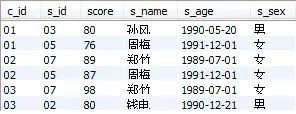# 23、统计各科成绩各分数段人数：课程编号,课程名称,[100-85],[85-70],[70-60],[0-60]及所占百分比

select a.c_id as '课程编号',course.c_name as '课程名称',
sum(level1) as '[100-85]人数', sum(level1)/count(1) as '[100-85]占比',
sum(level2) as '[85-70]人数', sum(level2)/count(1) as '[85-70]占比',
sum(level3) as '[70-60]人数', sum(level3)/count(1) as '[70-60]占比',
sum(level4) as '[0-60]人数', sum(level4)/count(1) as '[0-60]占比' from
(select *,
(case when score between 85 and 100 then 1 else 0 end) as 'level1',
(case when score between 70 and 84 then 1 else 0 end) as 'level2',
(case when score between 60 and 69 then 1 else 0 end) as 'level3',
(case when score between 0 and 59 then 1 else 0 end) as 'level4'
from score) a
left join course on a.c_id=course.c_id
group by a.c_id;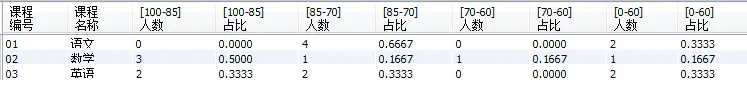# 24、查询学生平均成绩及其名次

select a.*,@rank:=@rank+1 as rank from
(select s_id,avg(score) as '平均成绩' from score
group by s_id order by avg(score) desc) a,
(select @rank:=0) b;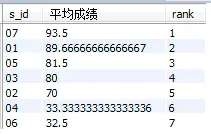# ****25、查询各科成绩前三名的记录

select a.c_id,a.s_id,a.score
from score a
where (select count(b.s_id) from score b where a.c_id=b.c_id and a.score<b.score)<3
group by a.c_id,a.s_id;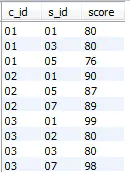# 26、查询每门课程被选修的学生数

select c_id,count(s_id) as '选修人数'
from score group by c_id;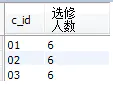# 27、查询出只有两门课程的全部学生的学号和姓名

select student.* from
(select s_id from score
group by s_id having count(c_id)=2) a
left join student on a.s_id=student.s_id;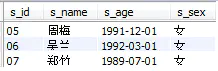# 28、查询男生、女生人数

select s_sex as '性别',count(1) as '人数'
from student group by s_sex;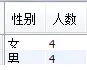# 29、查询名字中含有"风"字的学生信息

select * from student
where s_name like '%风%';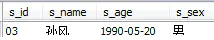# 30、查询同名同姓学生名单，并统计同名人数

select s_name,num as '同名人数' from
(select *,count(s_id)-1 as num
from student group by s_name) a;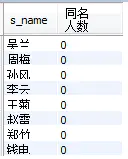# 31、查询1990年出生的学生名单(注：Student表中Sage列的类型是datetime)

select s_name from student where year(s_age)='1990';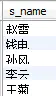# 32、查询每门课程的平均成绩，结果按平均成绩降序排列，平均成绩相同时，按课程编号

select c_id,avg(score) as '平均成绩'
from score group by c_id
order by 平均成绩 desc,c_id;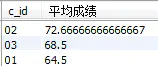# 33、查询平均成绩大于等于85的所有学生的学号、姓名和平均成绩

select a.s_id,s_name,avg_score from
(select s_id,avg(score) as avg_score from score
group by s_id having avg(score)>=85) a
left join student on a.s_id=student.s_id;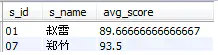image.png

# 34、查询课程名称为"数学"，且分数低于60的学生姓名和分数

select s_name,c_name,score from total
where c_name='数学' and score<60;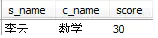# 35、查询所有学生的课程及分数情况

select s_id,
sum(case when c_id='01' then score else 0 end) as '语文',
sum(case when c_id='02' then score else 0 end) as '数学',
sum(case when c_id='03' then score else 0 end) as '英语'
from total
group by s_id;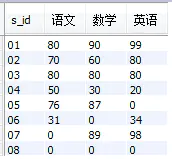# 36、查询任何一门课程成绩在70分以上的姓名、课程名称和分数

select s_name,c_name,score
from total where score>70;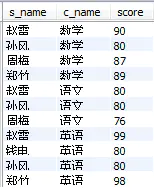# 37、查询不及格的课程

select score.c_id,course.c_name,score
from score left join course
on score.c_id=course.c_id
where score<60;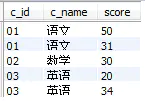# 38、查询课程编号为01且课程成绩在80分以上的学生的学号和姓名

select student.s_id,s_name from student
right join score on student.s_id=score.s_id
where c_id='01' and score>80;


# 39、求每门课程的学生人数

select c_id,count(1) as '选课人数'
from score group by c_id;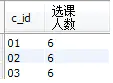# 40、查询选修"张三"老师所授课程的学生中，成绩最高的学生信息及其成绩

select student.*,a.score from
(select s_id,score
from total where t_name='张三'
order by score desc limit 1) a
left join student on a.s_id=student.s_id;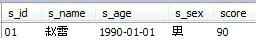# 41、查询不同课程成绩相同的学生的学生编号、课程编号、学生成绩

select a.s_id,a.c_id,a.score
from score a,score b
where a.c_id=b.c_id and a.s_id<>b.s_id and a.score=b.score;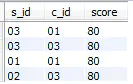# 42、查询每门功成绩最好的前两名

(select c_id,s_id from score where c_id='01' order by score limit 2)
union
(select c_id,s_id from score where c_id='02' order by score limit 2)
union
(select c_id,s_id from score where c_id='03' order by score limit 2);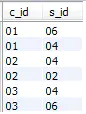# 43、统计每门课程的学生选修人数（超过5人的课程才统计）。要求输出课程号和选修人数，查询结果按人数降序排列，若人数相同，按课程号升序排列

select c_id,count(s_id) as 选修人数 from score
group by c_id having 选修人数>5
order by 选修人数 desc,c_id;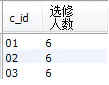# 44、检索至少选修两门课程的学生学号

select s_id from score group by s_id having count(c_id)>=2;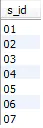# 45、查询选修了全部课程的学生信息

select * from student
where s_id in
(select s_id from score
group by s_id having count(c_id)=(select count(*) from course));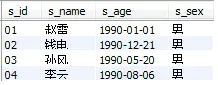# 46、查询各学生的年龄

select s_id,s_name,(year(now())-year(s_age)) as '年龄' from student;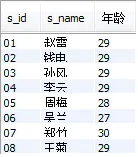# ***47、查询本周过生日的学生

select s_name,s_age from student
where date_format(s_age,'2019-%m-%d')


# 48、查询下周过生日的学生

select s_name,s_age from student
where date_format(s_age,'2019-%m-%d')


# 49、查询本月过生日的学生

select s_name,s_age from student
where date_format(s_age,'%m')=date_format(now(),'%m');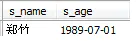（小白写这篇的时候是7月）

# 50、查询下月过生日的学生

select s_name,s_age from student
where date_format(s_age,'%m')=date_format(now(),'%m')+1;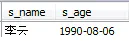©️2019 CSDN 皮肤主题: 书香水墨 设计师: CSDN官方博客